Chi-square/Fisher’s Exact Test

Example Codes:

Independence Testing

A test of independence is a statistical test that determines whether two categorical variables associate with each other. Chi-square test and Fisher’s exact test, which apply to contingency tables, are common approaches of independence testing.

Fisher’s exact test is one of the exact tests, while chi-square test is based on approximation. When there are more than 20% of cells with < 5 expected frequencies, Fisher’s exact test is preferrable to chi-square test because applying approximation is inadequate.

If the corresponding p-value of the test statistic is less than the chosen significance level, then the association between the two variables is statistically significant.

Chi-square Test

Chi-square test is a non-parametric statistical hypothesis test. The null hypothesis is that the observed frequency is consistent with the expected frequency of certain events in a sample. If the frequency distribution of a categorical variable does not differ across groups from another categorical variable, the two variables can be concluded as independent.

The test statistic is: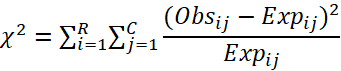Following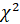distribution with degrees of freedom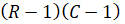and where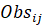is the observed frequency,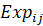is the expected count,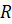is the number of rows of table and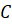is the number of columns.

Fisher’s Exact Test

Fisher’s Exact Test is based on a hypergeometric distribution of the counts in cells of the contingency table. A 2 x 2 contingency table is shown below:

 A Not A Total B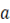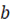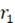Not B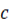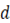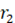Total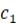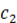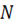The probability of obtaining such frequency distribution is: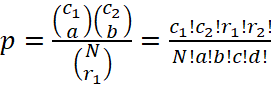Some statistical analysis software and packages, for example, SAS, supports Fisher’s exact test on general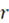xtables.

Example Code in SAS

DATA PERSONS ; INPUT GROUP \$ SUCCESS \$ @@;

DATALINES ;

DRUG NO DRUG NO DRUG NO DRUG YES

DRUG YES DRUG YES DRUG YES DRUG YES

DRUG YES DRUG YES

PLACEBO NO PLACEBO NO PLACEBO YES PLACEBO YES

PLACEBO YES PLACEBO YES PLACEBO YES PLACEBO YES

PLACEBO YES PLACEBO YES

RUN ;

PROC FREQ DATA = PERSONS ;

TABLES GROUP * SUCCESS/ NOPERCENT NOCOL NOROW

CHISQ FISHER EXPECTED ;

RUN ;

Example Code in R

i# input data

success <- c("No", "No", "No", "Yes", "Yes", "Yes", "Yes", "Yes", "Yes", "Yes",

"No", "No", "Yes", "Yes", "Yes", "Yes", "Yes", "Yes", "Yes", "Yes")

group <- c("drug", "drug", "drug", "drug", "drug", "drug", "drug", "drug",

"drug", "drug", "placebo", "placebo", "placebo", "placebo",

"placebo", "placebo", "placebo", "placebo", "placebo", "placebo")

# create a dataframe

df <- data.frame(success, group)

# contingency table

table(df)

# compute expected frequencies on the contingency table

xsq <- chisq.test(df\$success, df\$group)

xsq\$expected

# 50% of cells have expected counts less than 5. Use fisher's exact test

fisher.test(df\$success, df\$group)

References

1.     Kim H. Y. (2017). Statistical notes for clinical researchers: Chi-squared test and Fisher's exact test. Restorative dentistry & endodontics, 42(2), 152–155. https://doi.org/10.5395/rde.2017.42.2.152

2.     Hoffman, J. I. E. (2015). Biostatistics for Medical and Biomedical Practitioners. Academia Press. https://doi.org/10.1016/B978-0-12-802387-7.00013-5